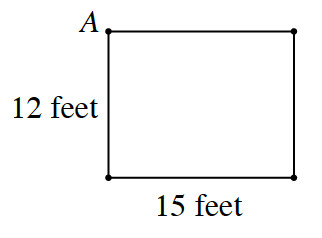### Home > CC2 > Chapter 4 > Lesson 4.2.4 > Problem4-64

4-64.

CECIL’S LATEST TRICKTo amaze the audience, Cecil set up four tightropes in a rectangle, each connected at a pole as shown in the diagram at right. A ladder down from the ropes is located at point $A$Homework Help ✎

1. If Cecil starts at point $A$ and must travel completely around the rectangle, how far must Cecil travel?

The distance Cecil must travel is the perimeter of the rectangle made by the four tightropes.

$54$ feet

2. How can Cecil’s distance be represented by an expression?

Perimeter can be written as $2(\text{width})+2(\text{length})$. Use this expression, substituting the appropriate values for ''width'' and ''length.''